# Lecture 19

## Trees

MCS 275 Spring 2022
David Dumas

### Lecture 19: Trees

Course bulletins:

• Project 2 due 6pm central Friday. Autograder open.

## Project 2 advice, part I

One way to write splittings and splittings_iterative is to use the fact that a splitting of n can be turned into a splitting of n+1 in two ways.

## Project 2 advice, part II

I think the easiest way to write splittings_distinct is to first write splittings_distinct_into and then have splittings_distinct call it.

(e.g. splittings_distinct(5) is the same thing as splittings_distinct_into(5,[1,2,3,4,5])

### Recently

We've talked about some recursive algorithms

### Next

Recursive data structures!

## Algorithm

A sequence of instructions that accomplishes a certain computation (e.g. find the square root of $n$) or solves a class of problems (e.g. given a list, find its largest element).

## Graphs

In mathematics, a graph is a collection of nodes (or vertices) and edges (which join pairs of nodes).

## Connectivity

A graph is connected if every pair of nodes can be joined by at least one path.

## Connectivity

A graph is connected if every pair of nodes can be joined by at least one path.

## Tree

A tree is a graph where every pair of nodes can be joined by exactly one path (no more, no less).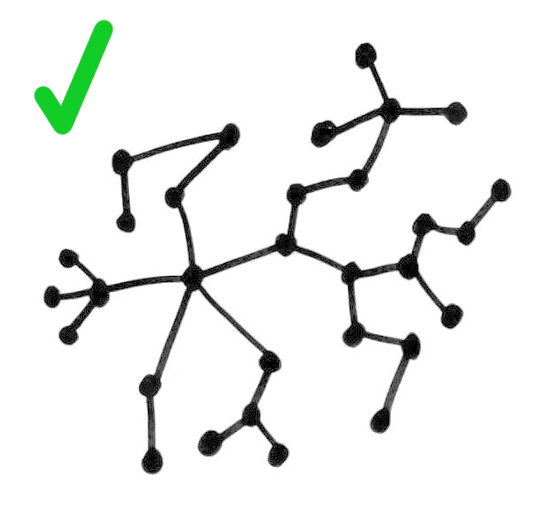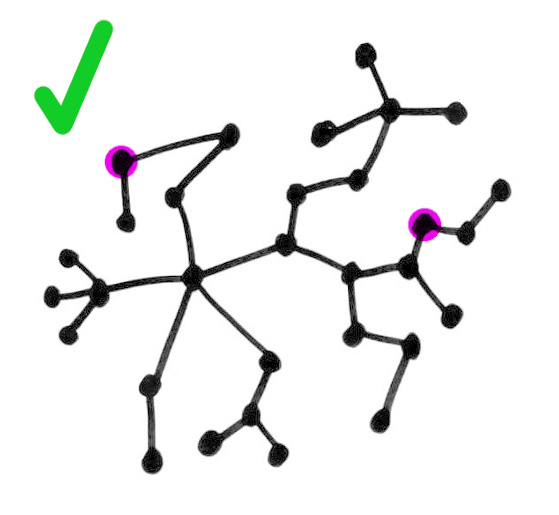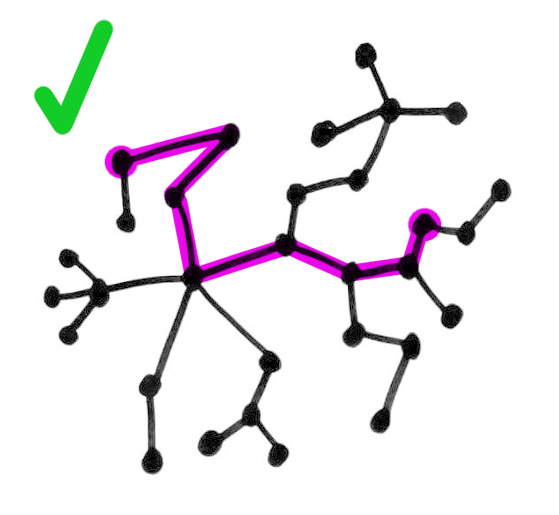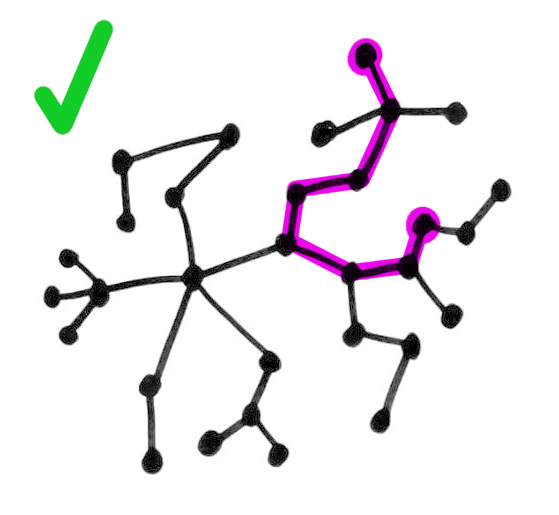## Tree

A tree is a graph where every pair of nodes can be joined by exactly one path (no more, no less).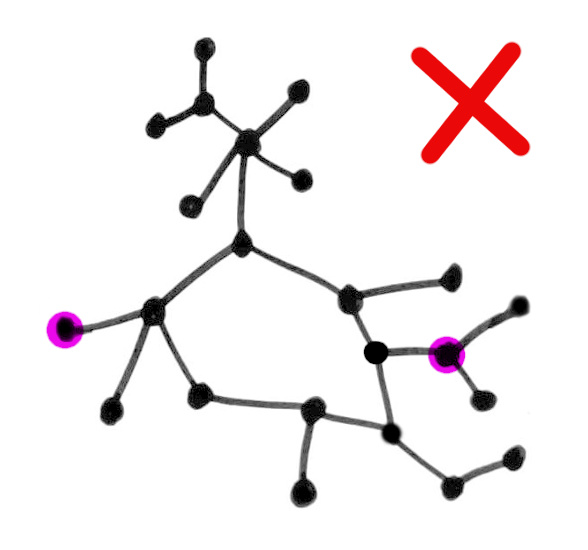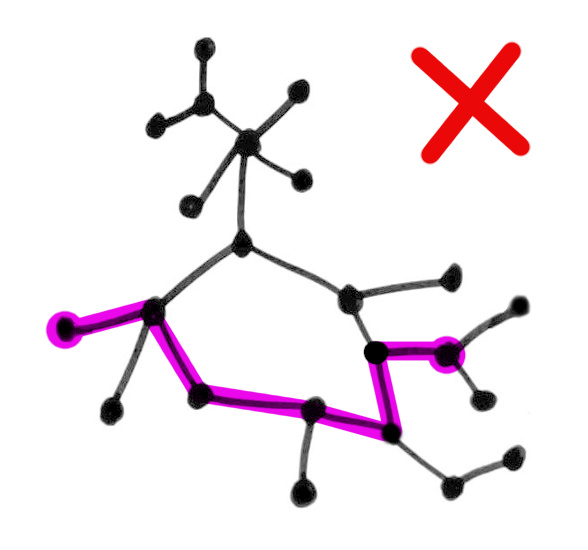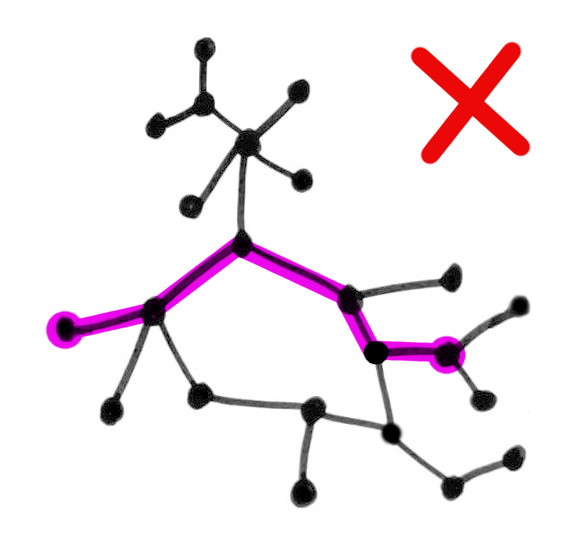Equivalently, a tree is a connected graph with no loops.

Equivalently, a tree is a connected graph that becomes disconnected if any edge is removed.

(Exercise: Prove this is an equivalent definition!)

## Example

The random mazes produced by maze.PrimRandomMaze(...) can be seen as trees, with the nodes being the open squares and edges between nodes if the corresponding squares share an edge.

## Roots and directions

The trees considered in CS usually have one node distinguished, called the root.

There's nothing special about the root except that it is labeled as such. Any node of a tree could be chosen to be its root node.

Such rooted trees are usually drawn with the root at top

and vertices farther from the root successively lower.

Such diagrams look like trees in the natural world.

## That maze again

Choosing a root lets us orient all of the edges so they point away from it.

Hence the usual way of drawing a tree will have these arrows pointing downward.

Each node (except the root) has an incoming edge, from its parent (closer to the root).

Each node may have one or more outgoing edges, to its children (farther from the root).

## Binary trees

In CS, a binary tree is a (rooted) tree in which every node has ≤ 2 children, labeled "left" and "right".

Horizontal relative position is used to indicate this labeling, rather than explicitly writing it on the edges.

## Binary trees

In CS, a binary tree is a (rooted) tree in which every node has ≤ 2 children, labeled "left" and "right".

Horizontal relative position is used to indicate this labeling, rather than explicitly writing it on the edges.

## Representation

How can we store a tree in Python?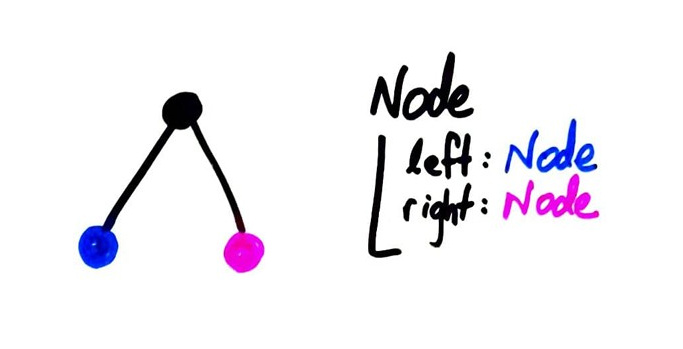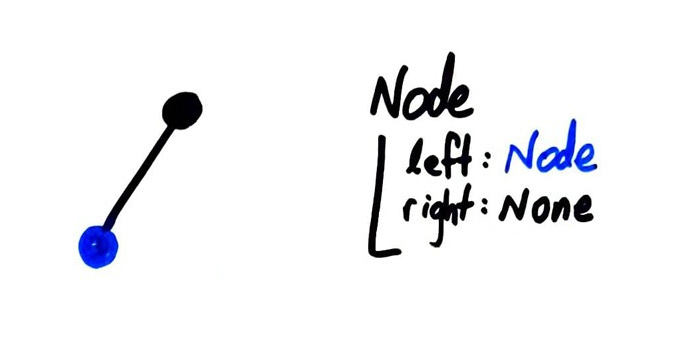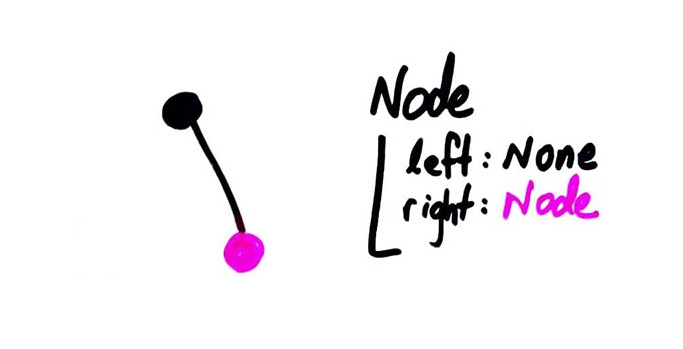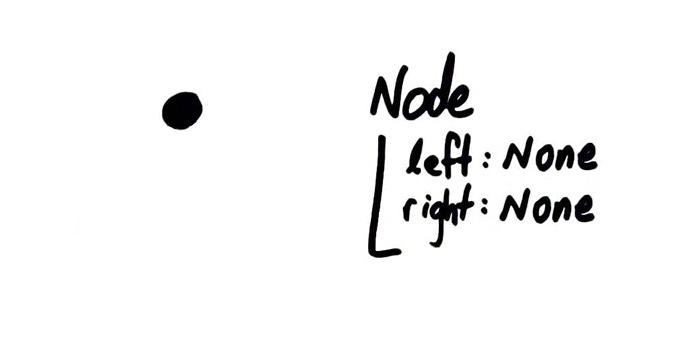Make a class Node, with attribues left and right that can be None or other Node objects.

## Why?

We can also store additional information in the nodes of a binary tree. If present, this is called the key or value or cargo of a node.

This turns out to be a very efficient data structure for many purposes. A lot of data can be accessed in a few steps from the root node.

## Binary search tree

Stores numbers or other objects allowing comparison as node values. Enforce the rule: Anything in the subtree to the left is smaller or equal. Anything in the subtree to the right is greater.

This allows a natural way to check if a value is present with a game of "too high / too low".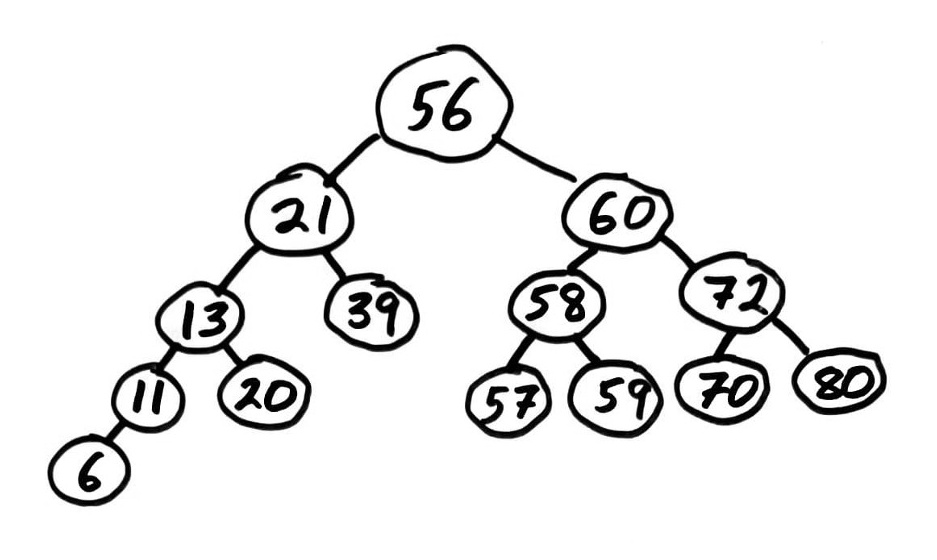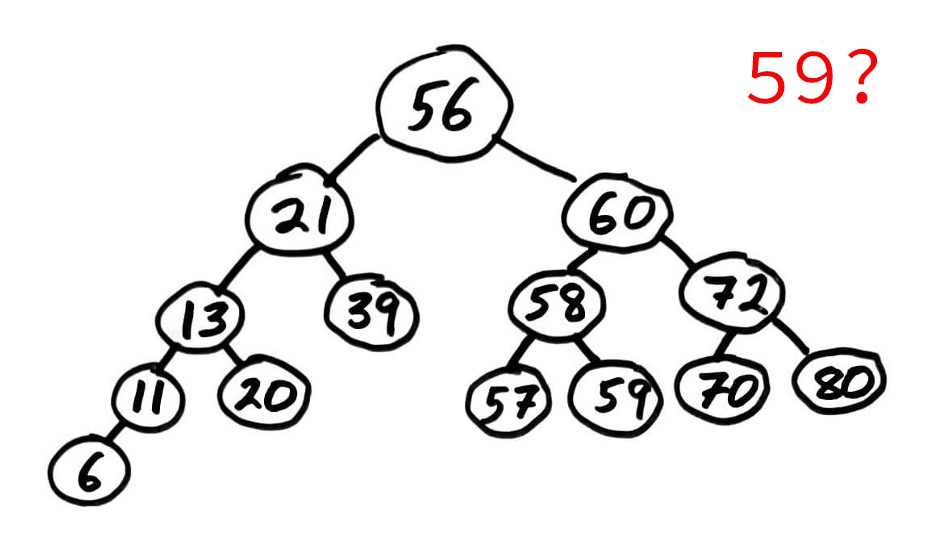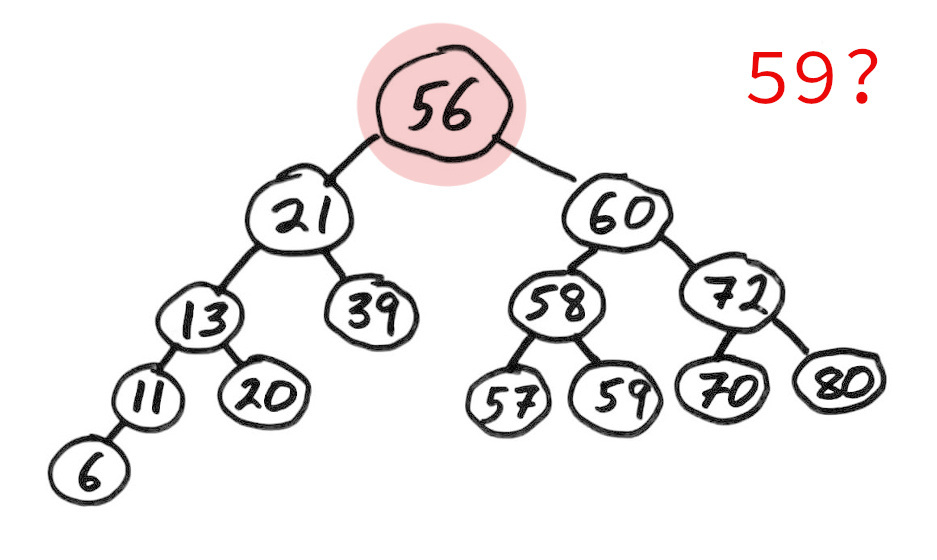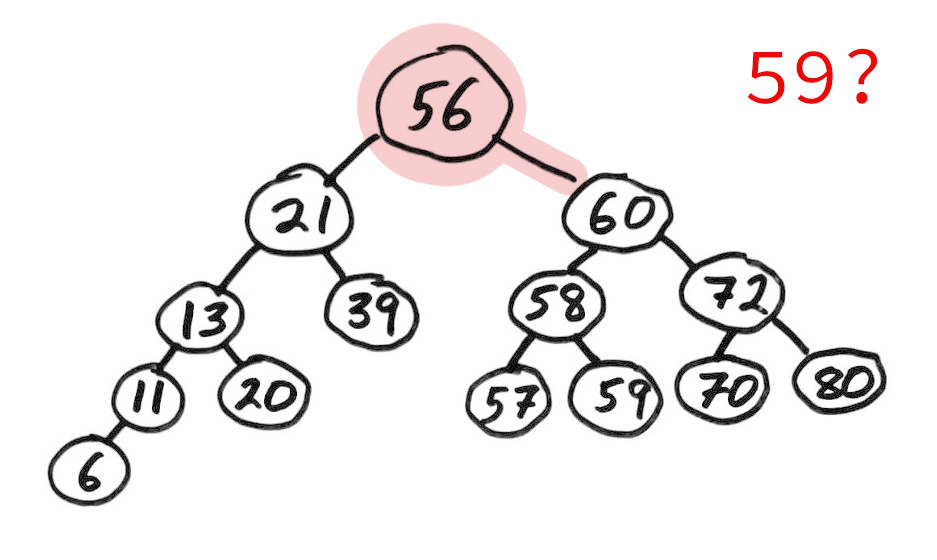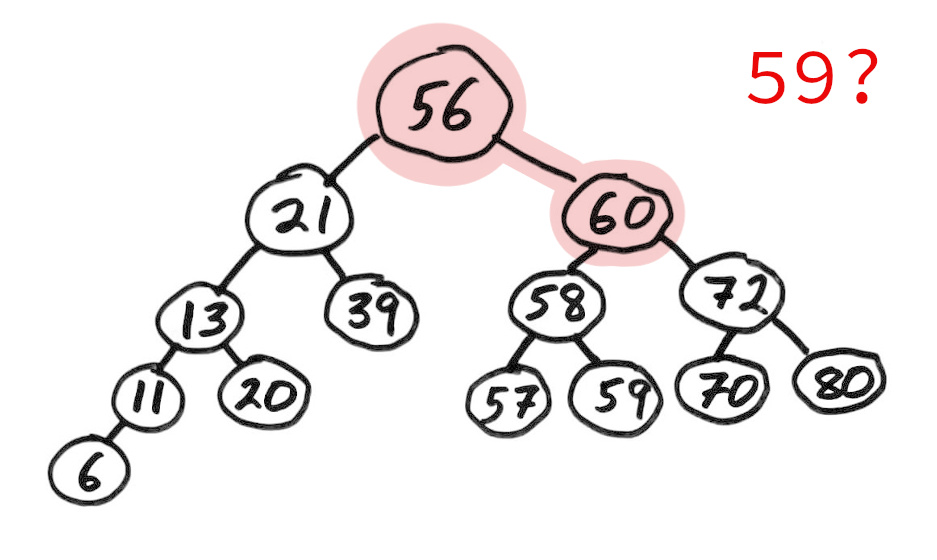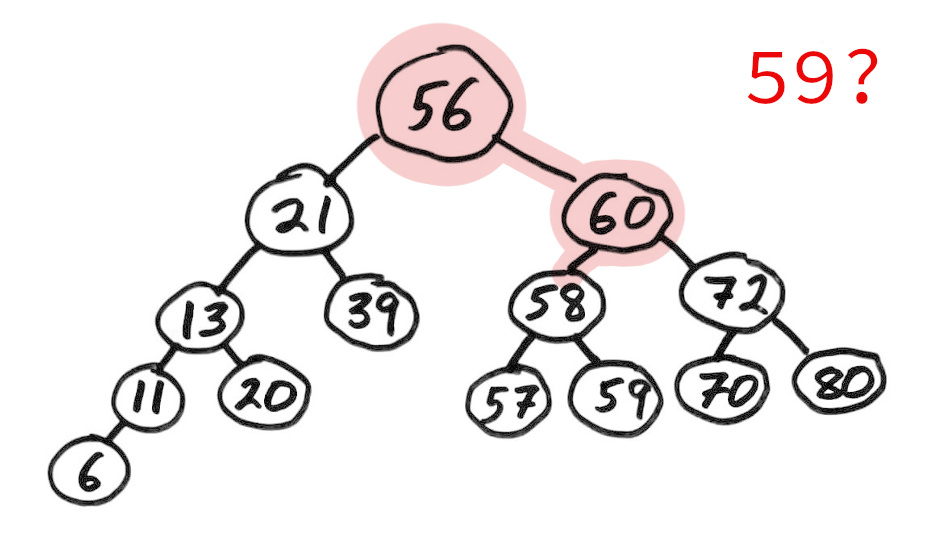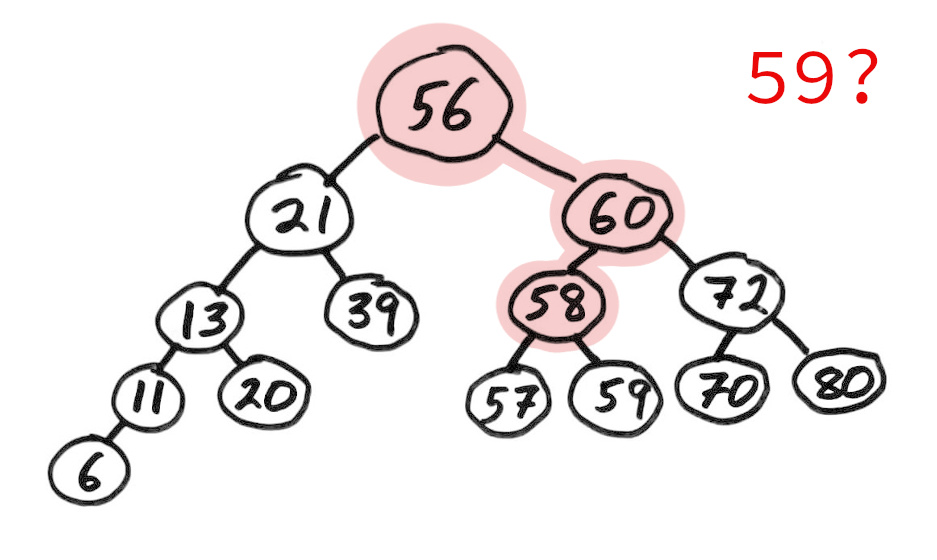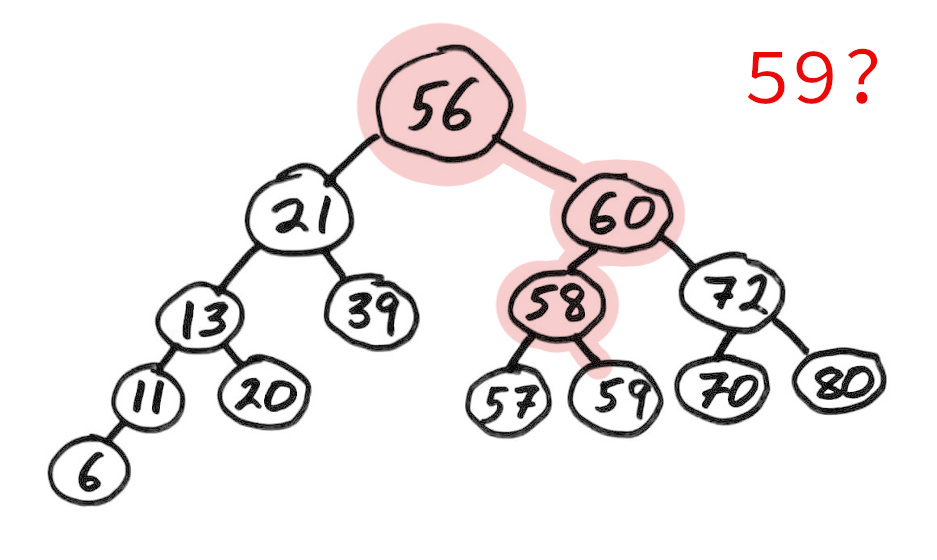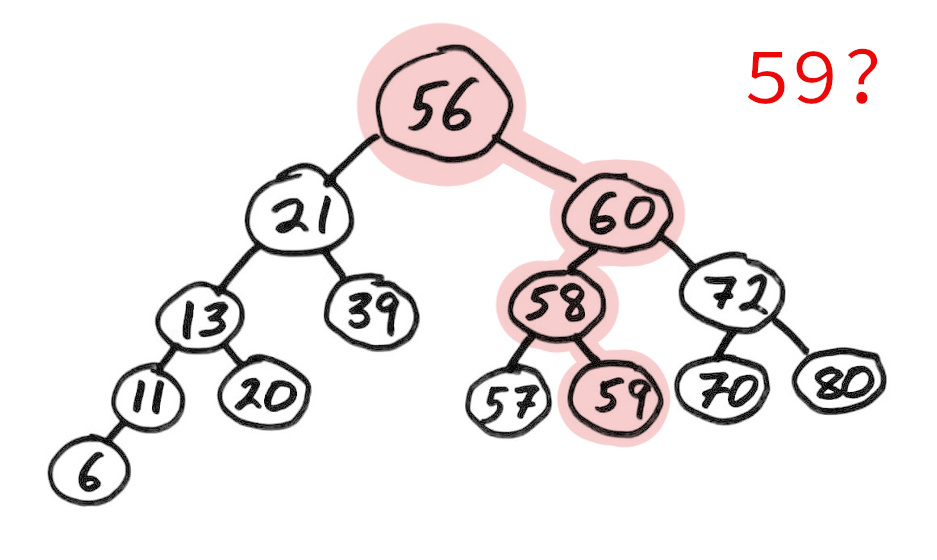### Revision history

• 2022-02-23 Initial publication